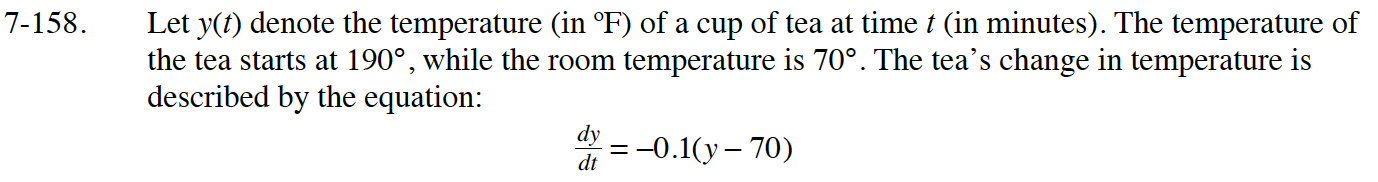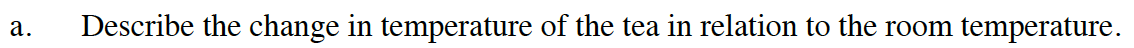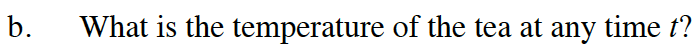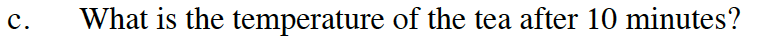Home > APCALC > Chapter 7 > Lesson 7.3.5 > Problem7-158

7-158.

Let $y(t)$ denote the temperature (in $ºF$) of a cup of tea at time t (in minutes). The temperature of the tea starts at $190°$, while the room temperature is $70°$. The tea’s change in temperature is described by the equation: Homework Help ✎

$\frac { d y } { d x }= −0.1(y − 70)$

1. ​Describe the change in temperature of the tea in relation to the room temperature.

2. What is the temperature of the tea at any time t?

3. What is the temperature of the tea after $10$ minutes?As time goes on, will the tea ever become colder than the room temperature?Implicitly integrate to find y(t).

Find the value of 'C' by evaluating the initial condition: when t = 0, y = 190.

y = 70 + 120e−0.1ty(10) = ________________